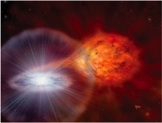Search:# Burst Analysis

Here we describe the analysis procedures for the bursts incorporated into the sample.

## BeppoSAX Wide Field Camera

The burst search was carried out as follows. We estimate the average flux and the standard deviation ($\sigma$) for each orbit (about 60~min of net exposure time). If a bin is at least 4$\sigma$ above the average flux we mark this as a candidate burst. We estimate that we have, on average, only one false peak triggered as a burst per observation. A triggered bin is visually approved as a burst if the shape of the lightcurve around the bin can be described by a fast rise and exponential decay. If needed, this is done at 1 s time resolution. Thus, we detect all bursts with e-folding times $\gtrsim2$~s and peak fluxes in excess of 0.5~Crab.

The WFCs detected 2202 type-I X-ray bursts from 54 LMXBs. The most prolific bursters in this data set are GX 354-0 (439 bursts), KS 1731-26 (343) and GS 1826-24 (271).

The time-resolved spectroscopy was carried out as follows.

1. The full-bandpass time profiles were fitted by an exponential function to determine the start time, the peak time, the exponential e-folding decay time over the complete bandpass, the peak flux (in ${\mathrm count\,s}^{-1}\,{\mathrm cm}^{-2}$) and the background flux. In general 15 s of pre-burst data were included and all data between the peak time and the time when the burst flux decayed by a factor of 5.
2. Time intervals were determined for which spectra would be extracted. The intervals were set by the requirement that the significance be 10. Experience showed that this would reveal spectra that had sufficient quality to allow a meaningful fit with a black body model.
3. Spectra were extracted for all burst time intervals, as well as for the complete observation that contained the burst. The latter spectra were normalized to match the background flux determined from the time profile fit. The spectra were extracted by generating for each energy channel and time interval an reconstructed image, fitting each image with a theoretical point spread function (PSF) leaving free the flux in the PSF and background flux, but keeping fixed the position to the value found from fitting the PSF for the whole burst and all energy channels. Response matrices were generated for every burst separately. These are mostly dependent on FOV position, WFC unit and time of observation.
4. All burst spectra were modeled by an absorbed black body model (model {\tt wabs*bbodyrad} in XSPEC), after being background subtracted with the scaled-up persistent spectrum determined in 3). The values for the equivalent hydrogen column density $N_{\rm H}$ were fixed to values that were found in the literature or determined from high-quality data found elsewhere encompassing $<$2 keV photon energies), see Table ?. The model fits yield bolometric flux, black body temperature and emission area (in terms of the radius of a sphere with that area) as a function of time.

Fluences were calculated by integrating all flux measurements up to the last spectroscopic point, supplemented with an estimate of the fluence beyond that point as extrapolated by the exponential fit to the light curve of the complete bandpass.

For every observation the persistent spectrum as extracted in point 3 was modeled with a power law and thermal bremsstrahlung. Absorption was included, fixing $N_{\rm H}$ to the literature value. Those fit results are included in the database that yielded the best fit, including the 3-25 keV flux and $\chi^2_\nu$.

Jean in 't Zand 30.4.2011

## RXTE Proportional Counter Array

Where not explicitly stated, the data analysis procedures are as in Galloway et al. (2008). For those bursts for which suitable datamodes were available, time-resolved spectra in the range 2--60~keV covering the burst duration were extracted on intervals beginnng at 0.25 s during the burst rise and peak. The bin size was gradually increased into the burst tail to maintain roughly the same signal-to-noise level. A spectrum taken from a 16-s interval prior to the burst was adopted as the background. % The {\it RXTE}\/ Proportional Counter Units (PCUs) are subject to a short ($\approx 10\ \mu$s) interval of inactivity following the detection of each X-ray photon. This deadtime'' reduces the detected count rate below what is incident on the detector (by approximately 3\% for an incident rate of 400~count~s$^{−1}$~PCU$^{−1}$). We corrected each spectrum for deadtime by calculating an effective exposure, depending upon the measured count rate, which takes into account the deadtime fraction.

We re-fit the spectra over the energy range 2.5--20~keV using the revised PCA response matrices, v11.7\footnote{see \url{ http://www.universe.nasa.gov/xrays/programs/rxte/pca/doc/rmf/pcarmf-11.7 } } and adopted the recommended systematic error of 0.5\%. The fitting was undertaken using {\sc XSpec } version 12. In order to accommodate spectral bins with low count rates, we adopted Churazov weighting. % We modelled the effects of interstellar absorption, using a multiplicative model component ({\tt wabs } in {\sc XSpec}), with the column density $n_{\rm H}$ frozen at insert details here. In the original analysis carried out by \cite{bcatalog}, the neutral absorption was determined separately for each burst, from the mean value obtained for spectral fits carried out with the $n_{\rm H}$ value free to vary. This has a negligible effect on the burst flux, but can introduce spurious burst-to-burst variations in the blackbody normalisation.

Duncan Galloway 24.9.2014